﻿ The ‘<i>Yard Stick</i>’ to Interpret the Entropy of Activation in Chemical Kinetics: A Physical-Organic Chemistry ExercisePublications are Open
Access in this journal
Article Versions
Export Article
• Normal Style
• MLA Style
• APA Style
• Chicago Style
Research Article
Open Access Peer-reviewed

### The ‘Yard Stick’ to Interpret the Entropy of Activation in Chemical Kinetics: A Physical-Organic Chemistry Exercise

World Journal of Chemical Education. 2018, 6(1), 78-81. DOI: 10.12691/wjce-6-1-12
Published online: January 27, 2018

### Abstract

No physical or physical-organic chemistry laboratory goes without a single instrument. To measure conductance we use conductometer, pH meter for measuring pH, colorimeter for absorbance, viscometer for viscosity, potentiometer for emf, polarimeter for angle of rotation, and several other instruments for different physical properties. But when it comes to the turn of thermodynamic or activation parameters, we don’t have any meters. The only way to evaluate all the thermodynamic or activation parameters is the use of some empirical equations available in many physical chemistry text books. Most often it is very easy to interpret the enthalpy change and free energy change in thermodynamics and the corresponding activation parameters in chemical kinetics. When it comes to interpretation of change of entropy or change of entropy of activation, more often it frightens than enlightens a new teacher while teaching and the students while learning. The classical thermodynamic entropy change is well explained by Atkins in terms of a sneeze in a busy street generates less additional disorder than the same sneeze in a quiet library (Figure 1) . The two environments are analogues of high and low temperatures, respectively. In this article making use of Eyring equation a factor usually called ‘universal factor’ is derived and made use as a ‘yard stick’ to interpreting the change in entropy of activation for physical or physical-organic chemistry senior undergraduate and graduate students’ class-room.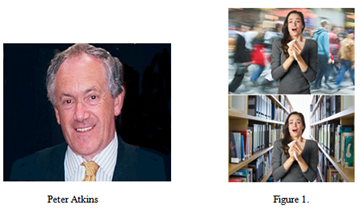### 1. Introduction

Thermodynamic properties like enthalpy, free energy and entropy of several thousands of organic and organometallic compounds were well documented and a very authoritative explanations and expert critical comments were offered 3, 4. As shown in the Figure 2, as an example taking any property (X = G free energy, or H enthalpy, or S entropy), thermodynamic and activation parameters could be distinguished between thermodynamics and kinetics. The nature of any property accompanied in chemical reactions in terms of energy considerations is nothing but an amalgamation of activation barrier (ΔX) and thermodynamic driving force (ΔXo). Marcus equation 5, 6, 7 is a successful treatise for treating kinetic data of electron transfer reactions to separate activation (ΔX) and thermodynamic quantities (ΔXo). The change in thermodynamic quantities could be interpreted in terms of classical thermodynamic principles. As an example the modern view of entropy is well described by Frank L. Lambert 8. In kinetics, activation parameters could be evaluated using both Arrhenius and Eyring equations. A one line argument about free energy of activation (∆G) and activation enthalpy (∆H) is just sufficed for interpretation from their sign and magnitude. But the interpretation of entropy of activation (∆S) is rather feared topic because of its complexity. Hence in this article a simple and lucid way of interpretation of entropy of activation is described.

### 2. Discussion

The effect of temperature on reaction rates is explained by Arrhenius equation 9, 10.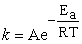(1)

or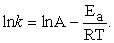(2)

The general way of determination of entropy of activation is from the intercept of an Arrhenius plot of log k versus 1/T.

Where k = rate constant,

Ea = activation energy,

T = absolute temperature,

R = gas constant and whose units are same that R,

and A = the pre-exponential factor.

The pre-exponential factor A will have the same units of k. And A is defined as the rate constant k of a reaction whose activation energy is zero or T is infinite 11.

From the familiar thermodynamic equation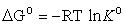(3)

one can write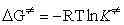(4)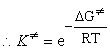(5)

where ∆G is the standard free energy of activation and is one of the activation parameters. From precepts of the transition state theory 12 it is known that the specific reaction rate or rate constant is given by the following equation: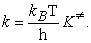(6)

Here K is the equilibrium constant of the reactants and the transition state starting with unit molar concentrations of reactants.

∴ From the equations 5 and 6, we get equation 7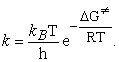(7)

From other familiar thermodynamic equation ∆Go = ∆Ho - T∆So one can write equation 8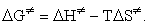(8)

Here ∆H and ∆S are standard molar enthalpy of activation and standard entropy of activation respectively. From equations 7 and 8 one can get equation 9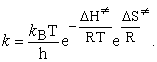(9)

This equation is known as famous Eyring equation 13.

And it is known that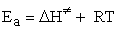or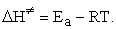(10)

Substituting ∆H in the equation 9, one can get the equation 11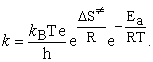(11)

Now comparing equations 1 and 11 one can conclude that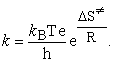(12)

Therefore for any activation ‘entropy neutral’ reaction (∆S = 0), that is the standard molar entropy of activated complex is equal to that of the reactants, then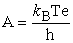(13)

‘A’ is called as the ‘universal factor’ and regarded as a component of pre-exponential factor of Arrhenius equation. It is nothing but the rate constant of a reaction at infinite temperature if T = ∞. Substituting the numerical values in the equation 13, and for all practical purposes to interpret the entropy of activation near the experimental temperature range, taking temperature equal to 298 K, A will be equal to1.7 X 1013 s-1. Then log A = 13.2. Therefore if the log A term from the intercept of Arrhenius plot is significantly less than this value the entropy of activation is negative. Therefore there will be loss in the entropy of activation during the formation of the activated complex. While if it is significantly greater than the value of 13.2, the formation of activated complex is accompanied by gain in entropy of activation hence ∆Swould be positive.

Therefore the termwould be taken as a ‘yard stick’ in the interpretation of entropy of activation beyond doubt. Some of the following examples would further enlighten the class-room atmosphere.

Example 1: Oxidation of α-hydroxy ethyl radical by nitrobenzenes via addition/elimination mechanism: As an example one with 4-nitrobenzonitrile.

The first case is the addition process 14 (scheme 1):

The intercept of Arrhenius plot for this reaction was found to be 10.9 which is significantly less than the value of 13.2. Correspondingly the entropy of activation for this addition reaction was found to be – 45 J (K mol)-1. The negative entropy of activation for this reaction is attributed to the larger fraction of electron transfer in the transition state which could be a contact ion pair type that is being solvated by surrounding water molecules and two reactant molecules becoming one transition state leading to decrease in translational degree of freedom. This gets further support from the Marcus slope 6 of 4.72 V-1 for this reaction 15 as compared to the value of 8.5 V-1 for a full electron transfer reaction between iron(II) and its substituted tris(1,10-phenanthroline) complexes by cerium(IV) 15, 16. However the change in entropy of activation assuming the reaction in gas-phase calculated using translational partition function was found to be – 64 J (K mol)-1. But this is a bit over simplification. However the negative change in entropy of activation could again be attributed to the loss in translational degree of freedom.

The second case is the heterolysis of the above adduct 17:

The intercept of Arrhenius plot for this reaction was found to be 10.2 which is significantly less than the value of 13.2. Again the entropy of activation for this heterolysis reaction was found to be – 58.5 J (K mol)-1. This very large negative entropy of activation was attributed to the extensive hydration of proton produced by the surrounding solvent water molecules based on the fact that hydration of one mole of proton by four water molecules leads to a decrease in entropy by 106 J (K mol)-1 18, 19. Hence there will be a very high loss in translational degree of freedom of solvent leading to negative entropy of activation. The change in the entropy of activation for the decomposition of the adduct using translational partition function was found to be 113 J (K mol)-1. But this is quite contrary to the value found experimentally. This could be due to the overwhelming large hydration of the proton produced during the heterolysis of the adduct. Hence the trend is unmistakable.

In addition to the above example, there were several other reactions involving various reducing radicals generated from alcohols and nucleic acid type bases like 6-methyl uracil, 6-methyl-i-cytosine and 6-methyl dihydrouracil with fourteen nitrobenzenes 14, 17, 20 and some solvolysis reactions of benzyl-gem-dichlorides, dibromides and diazides 21, 22, 23.

Some gas-phase reactions:

Example 1: Unimolecular gas-phase isomerization of bicycle[4.2.0]oct-7-ene 24 to give cyclooct-1,3-diene in the temperature range 508-558 K.

The Intercept from the Arrhenius plot was found to be 14.1s-1. At first sight this value is greater than the value of 13.2 obtained from the Universal Factor. Therefore the entropy of activation would be positive and using this value and equation 12, the entropy was found to be 11 J (K mol)-1. The possible structure of the transition state or activated complex would be: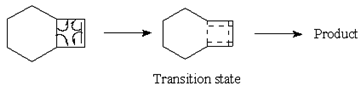If the possible uncertainty in measuring the entropy of activation is ± 10 J (K mol)-1, the value of 11 J (K mol)-1 is numerically very small leading to the conclusion that in the above reaction the entropy change is negligible. And this is reflected from the zero contribution of translational entropy as the molecular weights of the reactants, transition state and the products are the same. The equation for translational entropy is: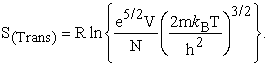In which the only variable is ‘m’ which is also constant in the above reaction. The small positive change in entropy could still be attributed to probably the strain of the cyclobutene ring in the reactant molecule that is relieved on the way to products.

The change in entropy of activation for the isomerization of iso-propenyl allyl ether was – 32 J (K mol)-1 which is obtained from the log A of 11.7 s-1 25. This was attributed to the formation a very rigid cyclic transition state as shown below: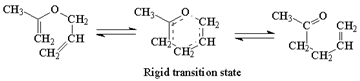The above are some of the putative examples which explain the title concept very well in several reactions to mention here but a few instances were explained in this article.

### References

  Peter Atkins, Entropy – a masterclass, Education in Chemistry, RSC Publications, Vol. 48, January issue, 2011. (http://www.rsc.org/Education/EiC/issues/2011January/EntropyAMasterclass.asp), © Hamish Kidd. In article View Article  Reproduced by permission of The Royal Society of Chemistry from Educ. Chem., 2011, (http://www.rsc.org/Education/EiC/issues/2011January/EntropyAMasterclass.asp), © Hamish Kidd. In article View Article  J. D. Cox and G. Pilcher, Thermochemistry of organic and organometllic compounds, Academic Press, New York (1970). In article View Article  D. R. Stull, E. F. Westrum and G. C. Sinke, The chemical thermodynamics of organic compounds, Wiley, New York (1969). In article View Article  R A Marcus, On the theory of oxidation-reduction reactions involving electron-transfer. I, J. Chem. Phys., 24, 966 (1956) In article View Article  On the theory of oxidation-reduction reactions involving electron transfer. v. comparison and properties of electrochemical and chemical rate constants, J. Phys. Chem., 67, 853, (1963), Marcus equation: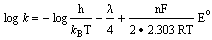the slope of log k vs Eo. In article View Article  See also for application of Marcus equation with a vareity of examples: The Marvelous Marcus equation: Distinguishing inner-sphere electron transfer reactions from outer-sphere electron transfer reactions: A one hour graduate class-room lecture, V. Jagannadham and R. Sanjeev, Bulgarian Chemical Communications, 42, 383 (2011). In article  Frank L. Lambert, A modern view of entropy, Chemistry (Khimiya, Bulgarian Journal of Education in Chemistry) 15, Issue 1 (2006) In article  S. Arrhenius, On the reaction rate of the inversion of non-refined sugar upon souring, Z. Phys. Chem., 4, 226 (1889). In article  M. Menzinger and R. Wolfgang, General account of the meaning and significance of the Arrhenius equation, Angew. Chem. Int. edn, 8, 438 (1969). In article View Article  V. Jagannadham, How do we introduce the Arrhenius factor (A) to graduate students? Creative Education, Published by Scientific Research Publishing Inc (USA) 1, 128 (2010). In article View Article  A. A. Frost and R. G. Pearson, Kinetics and Mechanism, Wiely Eastern, First reprint, New Delhi, page 71, (1971). In article  S. Glasstone, K. J. Laidler and H. Eyring, The theory of rate processes, McGraw-Hill (1941). In article  V. Jagannadham and S. Steenken, Reactivity of -heteroatom-substituted alkyl radicals with nitrobenzenes in aqueous solution: an entropy controlled electron transfer/addition mechanism, J. Am. Chem. Soc. 110, 2188 (1988). In article View Article  V. Jagannadham and R. Sanjeev, The Marvelous Marcus equation: Distinguishing inner-sphere electron transfer reactions from outer-sphere electron transfer reactions: A one hour graduate class-room lecture, Bulgarian Chemical Communications, vol. 42, page 383-394 (2011). In article  G. Dulz and N. Sutin, The Kinetics of the Oxidation of Iron(II) and its Substituted tris(1,10- phenanthroline) Complexes by Cerium(IV), Inorg. Chem., 2, 917 (1963). In article View Article  V. Jagannadham and S. Steenken, One-electron reduction of nitrobenzenes by α-hydroxyalkyl radicals via addition/elimination. An example of an organic inner-sphere electron-transfer reaction, J. Am. Chem. Soc, 106, 6542 (1984). In article View Article  H. –J. Buschmann, E. Dutkiewicz and W. Knoche, Study of reversible addition of water to carbonyl compounds, Ber. Bunsen-Ges. Phys. Chem., 86, 129, (1982). In article View Article  A. A. Frost and R. G. Pearson, Kinetics and Mechanism, Wiely, New York, page 300, (1961). In article  V. Jagannadham and S. Steenken, One-electron reduction of nitrobenzenes by hydroxyl and hydrogen radical adducts to 6-methyluracil and 6-methylisocytosine via electron transfer and addition/elimination: effect of substituents on rates and activation parameters for formation and heterolysis of nitroxyl-type tetrahedral intermediates. J. Phys. Chem. 92, 111 (1988). In article View Article  R. Sanjeev and V. Jagannadham, Substituent effects on the spontaneous cleavage of benzyl-gem-dichlorides in aqueous solution, Indian J. Chemistry, 41B, 2145 (2002). In article View Article  R. Sanjeev and V. Jagannadham, Substituent effects on the spontaneous cleavage of benzyl-gem-dibromides in aqueous solution, Indian J. Chemistry, 41A, 1841 (2002). In article View Article  V. Jagannadham, The carbocation lifetimes and entropy for addition of water to carbocations that are dependent on carbocation stability: The significance of entropy for nucleophilic solvation of α-azidobenzyl carbocations, Indian Academy of Sciences, 115, 41 (2003). In article View Article  G. R. Brandon, H. M. Frey and R. F. Skinner, Thermal isomerization of cyclobutenes. Part 8: Cis- and trans-1,2,3,4-tetramethylcyclobutene and bicyclo[4.2.0]oct-7-ene, Trans. Faraday Soc, 62, 1546 (1966). In article View Article  L. Stein and G. W. Murphy, Kinetics of the Rearrangement of Isopropenyl Allyl Ether, J. Am. Chem. Soc., 74, 1041 (1952). In article View ArticleThis work is licensed under a Creative Commons Attribution 4.0 International License. To view a copy of this license, visit http://creativecommons.org/licenses/by/4.0/